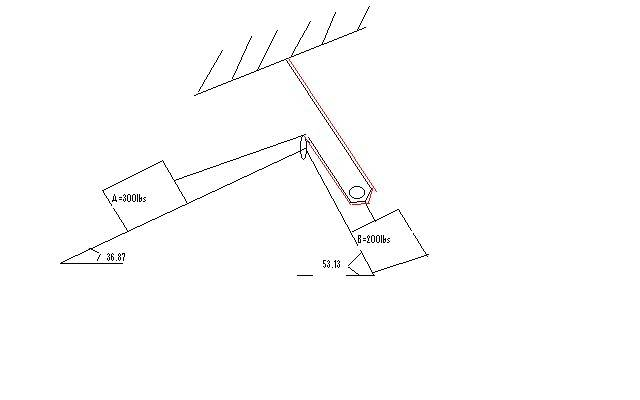# Newtons law of motion

apprentice213

## Homework Statement

Homework Statement
the blocks shown in the figure are connected by flexible, inextensible cords passing over frictionless pulleys. at A, the coefficient fiction is 0.30 while at B, it is 0.40. compute the magnitude and direction of the friction forces and each block.## Homework Equations

for block A=
T-mgsin53.13-Fmax=0
Fmax=0.3(300cos53.13)

for block B=
T-mgsin36.87-Fmax=0
Fmax=0.40(200cos36.87)

m(1)g-T=m(2)

## The Attempt at a Solution

for A:
Fmax=(o.30)(300cos53.13)
Fmax=54

T= 300(9.81)sin53.13
T=2354.4

for B :
Fmax=0.4(200cos36.87)
Fmax=54
T=200(9.81)sin36.87
T=1177.20

m1g-T=m2a
a=(2943-T)/200
a=244.3

## The Attempt at a Solution

danielakkerma
Hello!
Are the blocks to remain static? Does this question keep them stationary?
Daniel

danielakkerma
Then this
m1g-T=m2a
is impossible, since a = 0.
So try again, on that point alone.

apprentice213
sorry it is kenetic..

Last edited:
apprentice213
for A:

Fmax=μk(m1)gcos53.13
54=μk(m1)gcos53.13
μk=0.03

T-300(9.81)sin53.13-μk(300)9.81cos53.13=(300)a eq(1)
200g-T=200a eq(2)

subtitute (1) and (2) to find a

a=-3.79

for block B:

Fmax=μk(m2)gcos36.87
64=μk(m2)gcos36.87
μk=0.04

T-200(9.81)sin36.87-μk(200)9.81cos36.87=(200)a eq(1)
300g-T=300a eq(2)

subtitute (1) and (2)

to find a

a=0.2

AM i DOiNG RiGHT ?? please comment .. I am lack of knowledge ..

Last edited: